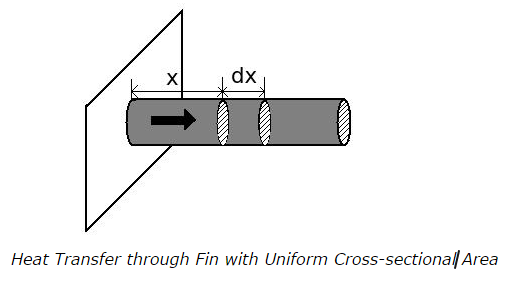# Heat Transfer Through Extended Surfaces (Fins) - MCQs

## Heat Transfer Through Extended Surfaces (Fins) - MCQs

1. What is the purpose of using fins in a particular heat transfer system?

a. to decrease rate of heat transfer
b. to increase rate of heat transfer
c. to maintain rate of heat transfer at a constant rate
d. cannot say

ANSWER: b. to increase rate of heat transfer

2. Temperature at the end tip of the fin having uniform cross-sectional area is

a. maximum
b. minimum
c. similar to the heat generation temperature
d. unpredictable

3. Consider that heat transfer is taking place through a fin having circular cross-sectional area, one dimensionally as shown in figure.The rate of heat transfer by conduction into a section at x is equal to

a. sum of rate of heat transfer by convection out of the element (x+dx) and heat transfer by convection from the surface between x to (x+dx)

b. sum of rate of heat transfer by conduction out of the element (x+dx) and heat transfer by conduction from the surface between x to (x+dx)

c. sum of rate of heat transfer by conduction out of the element (x+dx) and heat transfer by convection from the surface between x to (x+dx)

d. none of the above

ANSWER: c. sum of rate of heat transfer by conduction out of the element (x+dx) and heat transfer by convection from the surface between x to (x+dx)

4. In the process of heat transfer through extended surfaces or fins, the entire surface area is at

a. the same constant temperature
b. different temperatures
c. maximum base temperature
d. minimum temperature

5. An engine is fitted with pin fins having thermal conductivity k = 200 W/mK. The diameter and length of the fin is 2 cm and 50 cm respectively. Calculate the temperature at 10 cm from the fin base if fine base temperature is 500 0C and fin is in contact with air at 50 0C. Take h = 12 W/m2K
Consider that the fin is infinitely long

a. 80.67 0C
b. 100 0C
c. 85.67 0C
d. 185.67 0C